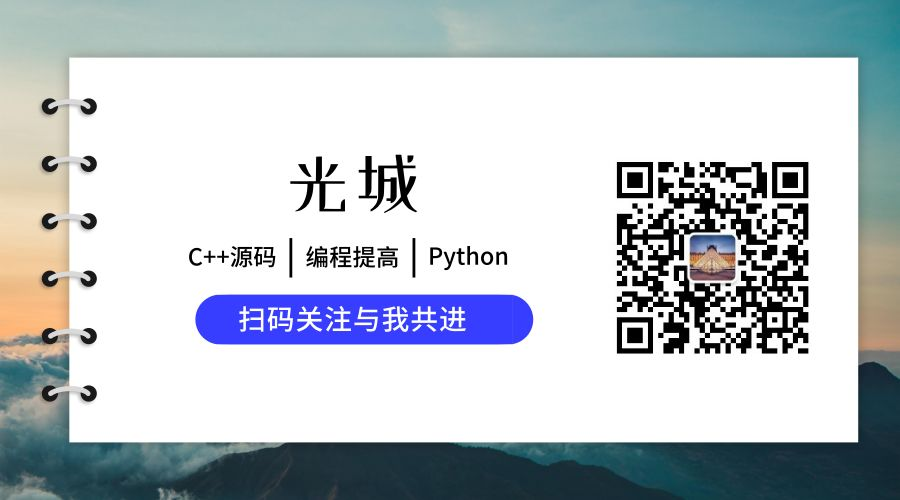# 【LeetCode每日一题】88. 合并两个有序数组

输入：nums1 = [1,2,3,0,0,0], m = 3, nums2 = [2,5,6], n = 3



class Solution {
public:
void merge(vector<int>& nums1, int m, vector<int>& nums2, int n) {
int mn = nums1.size();
while (mn) {
if (!m && n) {
nums1[--mn] = nums2[--n];
} else if (m && !n) {
break;
} else if (nums1[m-1] < nums2[n-1]) {
nums1[--mn] = nums2[--n];
} else {
nums1[--mn] = nums1[--m];
}
}
}
};


class Solution {
public:
void merge(vector<int>& nums1, int m, vector<int>& nums2, int n) {
int mn = nums1.size();
while (n) {
if (m==0 || nums1[m-1] < nums2[n-1]) {
nums1[--mn] = nums2[--n];
} else {
nums1[--mn] = nums1[--m];
}
}
}
};04-1364
07-31145
04-12115
04-12426
08-1279
03-24

### “相关推荐”对你有帮助么？

•非常没帮助
•没帮助
•一般
•有帮助
•非常有帮助被折叠的  条评论 为什么被折叠?到【灌水乐园】发言guangcheng0312q

¥2 ¥4 ¥6 ¥10 ¥20余额支付 (余额：-- )扫码支付获取中扫码支付点击重新获取扫码支付1.余额是钱包充值的虚拟货币，按照1:1的比例进行支付金额的抵扣。
2.余额无法直接购买下载，可以购买VIP、C币套餐、付费专栏及课程。余额充值